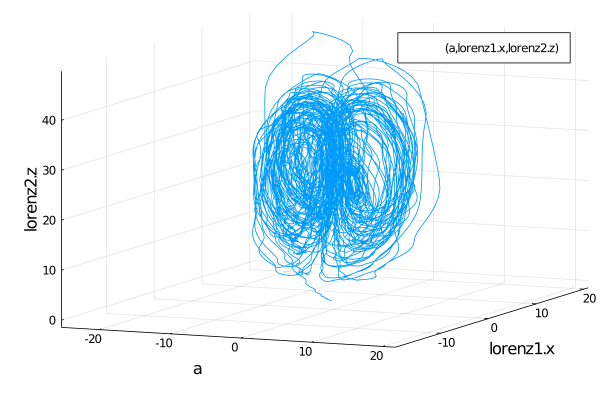# Component-Based Modeling with Ordinary Differential Equations

## Copy-Paste Example

Here is the complete example, with explanation to follow:

using ModelingToolkit, OrdinaryDiffEq

@parameters t σ ρ β
@variables x(t) y(t) z(t)
@derivatives D'~t

eqs = [D(x) ~ σ*(y-x),
D(y) ~ x*(ρ-z)-y,
D(z) ~ x*y - β*z]

lorenz1 = ODESystem(eqs,name=:lorenz1)
lorenz2 = ODESystem(eqs,name=:lorenz2)

@variables a
@parameters γ
connections = [0 ~ lorenz1.x + lorenz2.y + a*γ]
connected = ODESystem(connections,t,[a],[γ],systems=[lorenz1,lorenz2])

u0 = [lorenz1.x => 1.0,
lorenz1.y => 0.0,
lorenz1.z => 0.0,
lorenz2.x => 0.0,
lorenz2.y => 1.0,
lorenz2.z => 0.0,
a => 2.0]

p  = [lorenz1.σ => 10.0,
lorenz1.ρ => 28.0,
lorenz1.β => 8/3,
lorenz2.σ => 10.0,
lorenz2.ρ => 28.0,
lorenz2.β => 8/3,
γ => 2.0]

tspan = (0.0,100.0)
prob = ODEProblem(connected,u0,tspan,p)
sol = solve(prob,Rodas5())

using Plots; plot(sol,vars=(a,lorenz1.x,lorenz2.z))

## Generating ODESystems

First let's build an ODE model. To do this we start by defining some variables. In a differential equation system, we need to differentiate between our (dependent) variables and parameters. Therefore, we label them as follows:

using ModelingToolkit

@parameters t σ ρ β
@variables x(t) y(t) z(t)
@derivatives D'~t

Then we build the system:

eqs = [D(x) ~ σ*(y-x),
D(y) ~ x*(ρ-z)-y,
D(z) ~ x*y - β*z]

Each operation builds an Operation type, and thus eqs is an array of Operation and Variables. This holds a tree of the full system that can be analyzed by other programs. We can turn this into a ODESystem via:

sys = ODESystem(eqs)

This ODESystem can then be used to generate an ODEProblem by supplying the constructor with a map from the states of the system to their initial condition values and from the parameters of the system to their values. For example:

u0 = [x => 1.0
y => 0.0
z => 0.0]

p  = [σ => 10.0
ρ => 28.0
β => 8/3]
tspan = (0.0,100.0)
prob = ODEProblem(sys,u0,tspan,p;jac=true,sparse=true)

Note that the additional jac=true tells the system to symbolically generate an optimized Jacobian function to enhance the differential equation solvers, and sparse tells it to build the ODEProblem with all of the enhancements setup for sparse Jacobians.

## Building Component-Based Models

Now let's use ModelingToolkit to start building component-based models. Component-based models are compositions between submodels. This allows one to keep independently generated libraries of components intact and use them as the building blocks to construct more complicated models.

Let's define two interacting Lorenz equations. To do this, we will build two ODESystems from the equations we used in the first part:

lorenz1 = ODESystem(eqs,name=:lorenz1)
lorenz2 = ODESystem(eqs,name=:lorenz2)

Now let's define an interconnection between these ODE systems. Here we will define a new variable α which is defined by the interplay between these two models:

@variables a(t)
@parameters γ
connections = [0 ~ lorenz1.x + lorenz2.y + a*γ]
connected = ODESystem(connections,t,[a],[γ],systems=[lorenz1,lorenz2])

This ODESystem thus connects the two Lorenz systems and defines the dynamics of α according to the continuous algebraic equation, thus this is now a differential-algebraic equation (DAE) of 7 variables. We can then define the resulting ODEProblem and send it over to DifferentialEquations.jl:

u0 = [lorenz1.x => 1.0,
lorenz1.y => 0.0,
lorenz1.z => 0.0,
lorenz2.x => 0.0,
lorenz2.y => 1.0,
lorenz2.z => 0.0,
a => 2.0]

p  = [lorenz1.σ => 10.0,
lorenz1.ρ => 28.0,
lorenz1.β => 8/3,
lorenz2.σ => 10.0,
lorenz2.ρ => 28.0,
lorenz2.β => 8/3,
γ => 2.0]

tspan = (0.0,100.0)
prob = ODEProblem(connected,u0,tspan,p)
sol = solve(prob,Rodas5())

using Plots; plot(sol,vars=(a,lorenz1.x,lorenz2.z))